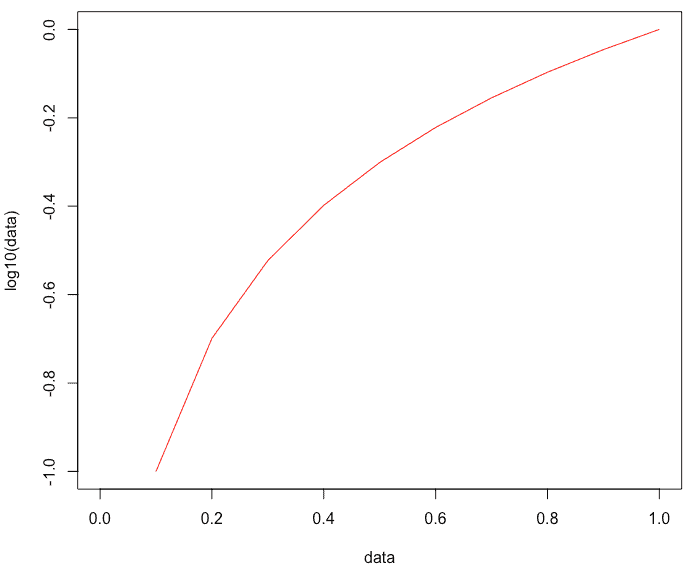# log10 in R: How to Use log10() Function in R Language

The log() function computes logarithms; by default, it calculates the natural logarithms. The log10() function computes common (for example, base 10) logarithms. The log2() function computes binary (i.e., base 2) logarithms. The common log(x, base) function computes logarithms with base.

## log10 in R

The log10() is an inbuilt R mathematical function that calculates the base 10 logarithms. The log() function calculates natural logarithms by default. The log2() method computes the logarithm of the given column in base 2.

### Syntax

``log10(x)``

### Parameters

It takes x as a column to calculate.

### Example

Let’s find the log10 of 5 using the log10() function.

``log10(5)``

#### Output

`` 0.69897``

Let’s find the log10 value of 0 and 1.

``````log10(0)
log10(1)``````

#### Output

`````` -Inf
 0``````

## Plot the log10() function in R

The most used plotting function in R programming is the plot() function. It is an inbuilt generic function with many methods called according to the type of object passed to the plot().

Open the rstudio and write the following code.

``````data <- seq(0, 1, by = 0.1)
plot(data, log10(data), typ = "l", col = "red")``````

#### Output## Find the log10() of the vector.

To find the log2 of the vector, pass the vector to the log10() function. Before that, you have to create a vector sequence if it is not available to you. Let’s create a vector sequence for our example.

``````vc <- (rep(1:5))
cat("The vector sequence is: ")
cat(vc, "\n")``````

#### Output

``The vector sequence is: 1 2 3 4 5``

To find the log10 of this vector, pass the sequence to a log10() function.

``````vc <- (rep(1:5))
cat("The vector sequence is: ")
cat(vc, "\n")
cat("Find the log10() of vector sequence is: ")
cat(log10(vc))``````

#### Output

``````The vector sequence is: 1 2 3 4 5
Find the log10() of vector sequence is: 0 0.30103 0.4771213 0.60206 0.69897
``````

That is it for log10() function in R language.

Categories R